Updating search results...

# 76 Results

View
Selected filters:
• Illustrative MathematicsUnrestricted Use
CC BY
Rating
0.0 stars

This task examines the ways in which the plane can be covered by regular polygons in a very strict arrangement called a regular tessellation. These tessellations are studied here using algebra, which enters the picture via the formula for the measure of the interior angles of a regular polygon (which should therefore be introduced or reviewed before beginning the task). The goal of the task is to use algebra in order to understand which tessellations of the plane with regular polygons are possible.

Material Type:
Activity/Lab
Provider:
Illustrative Mathematics
Provider Set:
Illustrative Mathematics
Author:
Illustrative Mathematics
01/21/2013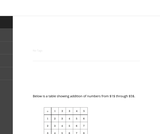Unrestricted Use
CC BY
Rating
3.0 stars

The purpose of this task is to study some patterns in a small addition table. Each pattern identified persists for a larger table and if more time is available for this activity students should be encouraged to explore these patterns in larger tables.

Material Type:
Activity/Lab
Provider:
Illustrative Mathematics
Provider Set:
Illustrative Mathematics
Author:
Illustrative Mathematics
08/15/2012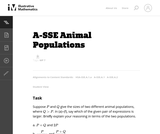Unrestricted Use
CC BY
Rating
0.0 stars

In this task students have to interpret expressions involving two variables in the context of a real world situation. All given expressions can be interpreted as quantities that one might study when looking at two animal populations.

Material Type:
Activity/Lab
Provider:
Illustrative Mathematics
Provider Set:
Illustrative Mathematics
Author:
Illustrative Mathematics
05/01/2012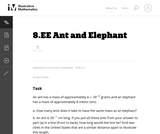Unrestricted Use
CC BY
Rating
0.0 stars

In this problem students are comparing a very small quantity with a very large quantity using the metric system. The metric system is especially convenient when comparing measurements using scientific notations since different units within the system are related by powers of ten.

Material Type:
Activity/Lab
Provider:
Illustrative Mathematics
Provider Set:
Illustrative Mathematics
Author:
Illustrative Mathematics
05/01/2012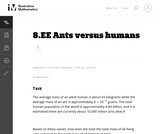Unrestricted Use
CC BY
Rating
0.0 stars

This task requires students to work with very large and small values expressed both in scientific notation and in decimal notation (standard form). In addition, students need to convert units of mass.

Material Type:
Activity/Lab
Provider:
Illustrative Mathematics
Provider Set:
Illustrative Mathematics
Author:
Illustrative Mathematics
08/21/2012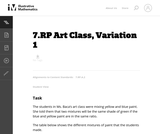Unrestricted Use
CC BY
Rating
0.0 stars

This this task about mixing paint requires students to graph ratios on a coordinate plane. It is a standard language in ratio problem.

Material Type:
Activity/Lab
Provider:
Illustrative Mathematics
Provider Set:
Illustrative Mathematics
Author:
Illustrative Mathematics
05/01/2012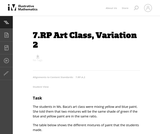Unrestricted Use
CC BY
Rating
0.0 stars

This this task about mixing paint requires students to graph ratios on a coordinate plane. It is a standard language in ratio problem.

Material Type:
Activity/Lab
Provider:
Illustrative Mathematics
Provider Set:
Illustrative Mathematics
Author:
Illustrative Mathematics
05/01/2012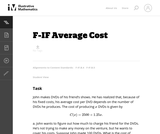Unrestricted Use
CC BY
Rating
0.0 stars

In this real world problem students solve questions based on the relationship between production costs and price.

Material Type:
Activity/Lab
Provider:
Illustrative Mathematics
Provider Set:
Illustrative Mathematics
Author:
Illustrative Mathematics
05/01/2012Unrestricted Use
CC BY
Rating
0.0 stars

This task provides a real world context for interpreting and solving exponential equations. There are two solutions provided for part (a). The first solution demonstrates how to deduce the conclusion by thinking in terms of the functions and their rates of change. The second approach illustrates a rigorous algebraic demonstration that the two populations can never be equal.

Material Type:
Activity/Lab
Provider:
Illustrative Mathematics
Provider Set:
Illustrative Mathematics
Author:
Illustrative Mathematics
05/01/2012Unrestricted Use
CC BY
Rating
0.0 stars

Material Type:
Activity/Lab
Provider:
Illustrative Mathematics
Provider Set:
Illustrative Mathematics
Author:
Illustrative Mathematics
05/01/2012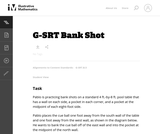Unrestricted Use
CC BY
Rating
0.0 stars

This task asks students to use similarity to solve a problem in a context that will be familiar to many, though most students are accustomed to using intuition rather than geometric reasoning to set up the shot.

Material Type:
Activity/Lab
Provider:
Illustrative Mathematics
Provider Set:
Illustrative Mathematics
Author:
Illustrative Mathematics
08/21/2012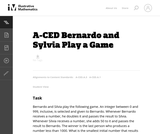Unrestricted Use
CC BY
Rating
0.0 stars

This task presents a simple but mathematically interesting game whose solution is a challenging exercise in creating and reasoning with algebraic inequalities. The core of the task involves converting a verbal statement into a mathematical inequality in a context in which the inequality is not obviously presented, and then repeatedly using the inequality to deduce information about the structure of the game.

Material Type:
Activity/Lab
Provider:
Illustrative Mathematics
Provider Set:
Illustrative Mathematics
Author:
Illustrative Mathematics
10/21/2013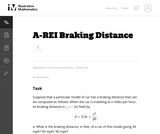Unrestricted Use
CC BY
Rating
0.0 stars

This task provides an exploration of a quadratic equation by descriptive, numerical, graphical, and algebraic techniques. Based on its real-world applicability, teachers could use the task as a way to introduce and motivate algebraic techniques like completing the square, en route to a derivation of the quadratic formula.

Material Type:
Activity/Lab
Provider:
Illustrative Mathematics
Provider Set:
Illustrative Mathematics
Author:
Illustrative Mathematics
05/01/2012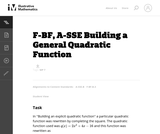Unrestricted Use
CC BY
Rating
0.0 stars

This task is for instructional purposes only and builds on ``Building an explicit quadratic function.''

Material Type:
Activity/Lab
Provider:
Illustrative Mathematics
Provider Set:
Illustrative Mathematics
Author:
Illustrative Mathematics
08/20/2012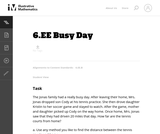Unrestricted Use
CC BY
Rating
3.0 stars

This task provides a good entry point for students into representing quantities in contexts with variables and expressions and building equations that reflect the relationships presented in the context.

Material Type:
Activity/Lab
Provider:
Illustrative Mathematics
Provider Set:
Illustrative Mathematics
Author:
Illustrative Mathematics
08/15/2012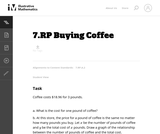Unrestricted Use
CC BY
Rating
0.0 stars

This is a task where it would be appropriate for students to use technology such as a graphing calculator or GeoGebra, making it a good candidate for students to engage in Standard for Mathematical Practice 5 Use appropriate tools strategically.

Material Type:
Activity/Lab
Provider:
Illustrative Mathematics
Provider Set:
Illustrative Mathematics
Author:
Illustrative Mathematics
05/01/2012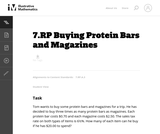Unrestricted Use
CC BY
Rating
0,0 stars

This task requires students to solves multistep ratio and percent problem.

نوع المادة:
Activity/Lab
Provider:
Illustrative Mathematics
Provider Set:
Illustrative Mathematics
المؤلف:
Illustrative Mathematics
05/01/2012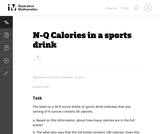Unrestricted Use
CC BY
Rating
0,0 stars

This problem involves the meaning of numbers found on labels. When the level of accuracy is not given we need to make assumptions based on how the information is reported. The goal of the task is to stimulate a conversation about rounding and about how to record numbers with an appropriate level of accuracy, tying in directly to the standard N-Q.3. It is therefore better suited for instruction than for assessment purposes.

نوع المادة:
Activity/Lab
Provider:
Illustrative Mathematics
Provider Set:
Illustrative Mathematics
المؤلف:
Illustrative Mathematics
01/05/2013Unrestricted Use
CC BY
Rating
0,0 stars

This task presents a real-world problem requiring the students to write linear equations to model different cell phone plans. Looking at the graphs of the lines in the context of the cell phone plans allows the students to connect the meaning of the intersection points of two lines with the simultaneous solution of two linear equations.

نوع المادة:
Activity/Lab
Provider:
Illustrative Mathematics
Provider Set:
Illustrative Mathematics
المؤلف:
Illustrative Mathematics
05/01/2012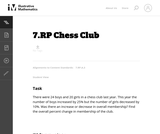Unrestricted Use
CC BY
Rating
0,0 stars

This problem includes a percent increase in one part with a percent decrease in the remaining and asks students to find the overall percent change. The problem may be solved using proportions or by reasoning through the computations or writing a set of equations.

نوع المادة:
Activity/Lab
Provider:
Illustrative Mathematics
Provider Set:
Illustrative Mathematics
المؤلف:
Illustrative Mathematics
05/01/2012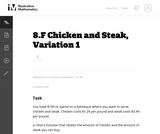Unrestricted Use
CC BY
Rating
0,0 stars

This task presents a real world situation that can be modeled with a linear function best suited for an instructional context.

نوع المادة:
Activity/Lab
Provider:
Illustrative Mathematics
Provider Set:
Illustrative Mathematics
المؤلف:
Illustrative Mathematics
05/01/2012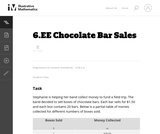Unrestricted Use
CC BY
Rating
0,0 stars

In this task students use different representations to analyze the relationship between two quantities and to solve a real world problem. The situation presented provides a good opportunity to make connections between the information provided by tables, graphs and equations.

نوع المادة:
Activity/Lab
Provider:
Illustrative Mathematics
Provider Set:
Illustrative Mathematics
المؤلف:
Illustrative Mathematics
08/08/2012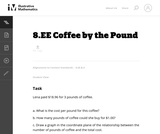Unrestricted Use
CC BY
Rating
0.0 stars

Students are exptected to identify the slope of the line with the unit rate in this real world problem.

Material Type:
Activity/Lab
Provider:
Illustrative Mathematics
Provider Set:
Illustrative Mathematics
Author:
Illustrative Mathematics
05/01/2012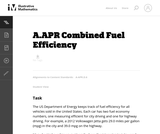Unrestricted Use
CC BY
Rating
0.0 stars

The primary purpose of this problem is to rewrite simple rational expressions in different forms to exhibit different aspects of the expression, in the context of a relevant real-world context (the fuel efficiency of of a car). Indeed, the given form of the combined fuel economy computation is useful for direct calculation, but if asked for an approximation, is not particularly helpful.

Material Type:
Activity/Lab
Provider:
Illustrative Mathematics
Provider Set:
Illustrative Mathematics
Author:
Illustrative Mathematics
01/25/2013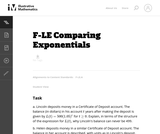Unrestricted Use
CC BY
Rating
0.0 stars

This task gives students an opportunity to work with exponential functions in a real world context involving continuously compounded interest. They will study how the base of the exponential function impacts its growth rate and use logarithms to solve exponential equations.

Material Type:
Activity/Lab
Provider:
Illustrative Mathematics
Provider Set:
Illustrative Mathematics
Author:
Illustrative Mathematics
05/01/2012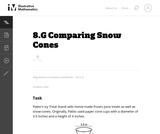Unrestricted Use
CC BY
Rating
0.0 stars

Students must calculate the volume of a cone in this real world task.

Material Type:
Activity/Lab
Provider:
Illustrative Mathematics
Provider Set:
Illustrative Mathematics
Author:
Illustrative Mathematics
05/01/2012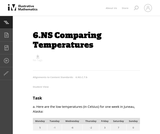Unrestricted Use
CC BY
Rating
0.0 stars

The purpose of the task is for students to compare signed numbers in a real-world context.

Material Type:
Activity/Lab
Provider:
Illustrative Mathematics
Provider Set:
Illustrative Mathematics
Author:
Illustrative Mathematics
07/23/2012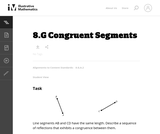Unrestricted Use
CC BY
Rating
0.0 stars

Students' first experience with transformations is likely to be with specific shapes like triangles, quadrilaterals, circles, and figures with symmetry. Exhibiting a sequence of transformations that shows that two generic line segments of the same length are congruent is a good way for students to begin thinking about transformations in greater generality.

Material Type:
Activity/Lab
Provider:
Illustrative Mathematics
Provider Set:
Illustrative Mathematics
Author:
Illustrative Mathematics
05/01/2012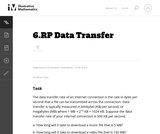Unrestricted Use
CC BY
Rating
0.0 stars

This task asks the students to solve a real-world problem involving unit rates (data per unit time) using units that many teens and pre-teens have heard of but may not know the definition for. While the computations involved are not particularly complex, the units will be abstract for many students.

Material Type:
Activity/Lab
Provider:
Illustrative Mathematics
Provider Set:
Illustrative Mathematics
Author:
Illustrative Mathematics
12/14/2012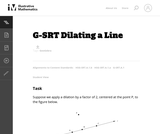Unrestricted Use
CC BY
Rating
0.0 stars

This task gives students the opportunity to verify that a dilation takes a line that does not pass through the center to a line parallel to the original line, and to verify that a dilation of a line segment (whether it passes through the center or not) is longer or shorter by the scale factor.

Material Type:
Activity/Lab
Provider:
Illustrative Mathematics
Provider Set:
Illustrative Mathematics
Author:
Illustrative Mathematics
05/01/2012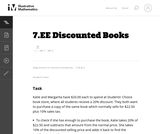Unrestricted Use
CC BY
Rating
0.0 stars

This purpose of this task is to help students see two different ways to look at percentages both as a decrease and an increase of an original amount. In addition, students have to turn a verbal description of several operations into mathematical symbols.

Material Type:
Activity/Lab
Provider:
Illustrative Mathematics
Provider Set:
Illustrative Mathematics
Author:
Illustrative Mathematics
05/01/2012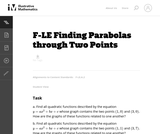Unrestricted Use
CC BY
Rating
0.0 stars

In this task students have the opportunity to construct linear and exponential functions, including arithmetic and geometric sequences, given a graph, a description of a relationship, or two input-output pairs (include reading these from a table).

Material Type:
Activity/Lab
Provider:
Illustrative Mathematics
Provider Set:
Illustrative Mathematics
Author:
Illustrative Mathematics
05/01/2012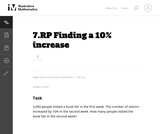Unrestricted Use
CC BY
Rating
0.0 stars

This real world problem is appropriate for mental mathematics and students should be encouraged to think through the solution mentally.

Material Type:
Activity/Lab
Provider:
Illustrative Mathematics
Provider Set:
Illustrative Mathematics
Author:
Illustrative Mathematics
05/01/2012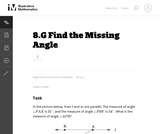Unrestricted Use
CC BY
Rating
0.0 stars

This task "Uses facts about supplementary, complementary, vertical, and adjacent angles in a multi-step problem to write and solve simple equations for an unknown angle in a figure (7.G.5)" except that it requires students to know, in addition, something about parallel lines, which students will not see until 8th grade.

Material Type:
Activity/Lab
Provider:
Illustrative Mathematics
Provider Set:
Illustrative Mathematics
Author:
Illustrative Mathematics
05/01/2012Unrestricted Use
CC BY
Rating
0.0 stars

In this task students are asked to write an equation to solve a real-world problem. There are two natural approaches to this task. In the first approach, students have to notice that even though there is one variable, namely the number of firefighters, it is used in two different places. In the other approach, students can find the total cost per firefighter and then write the equation.

Material Type:
Activity/Lab
Provider:
Illustrative Mathematics
Provider Set:
Illustrative Mathematics
Author:
Illustrative Mathematics
05/01/2012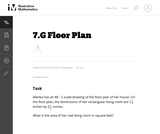Unrestricted Use
CC BY
Rating
0.0 stars

The purpose of this task is for students to translate between measurements given in a scale drawing and the corresponding measurements of the object represented by the scale drawing.

Material Type:
Activity/Lab
Provider:
Illustrative Mathematics
Provider Set:
Illustrative Mathematics
Author:
Illustrative Mathematics
05/01/2012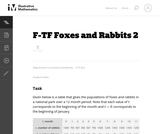Unrestricted Use
CC BY
Rating
0.0 stars

The example of rabbits and foxes was introduced in the task (8-F Foxes and Rabbits) to illustrate two functions of time given in a table. We are now in a position to actually model the data given previously with trigonometric functions and investigate the behavior of this predator-prey situation.

Material Type:
Activity/Lab
Provider:
Illustrative Mathematics
Provider Set:
Illustrative Mathematics
Author:
Illustrative Mathematics
08/29/2012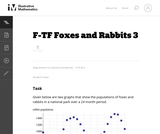Unrestricted Use
CC BY
Rating
0.0 stars

The example of rabbits and foxes was introduced in 8-F Foxes and Rabbits to illustrate two functions of time given in a table. The same situation was used in F-TF Foxes and Rabbits 2 to find trigonometric functions modeling the data in the table. The previous situation was somewhat unrealistic since we were able to find functions that fit the data perfectly. In this task, on the other hand, we do some legitimate modelling, in that we come up with functions that approximate the data well, but do not perfectly match, the given data.

Material Type:
Activity/Lab
Provider:
Illustrative Mathematics
Provider Set:
Illustrative Mathematics
Author:
Illustrative Mathematics
08/30/2012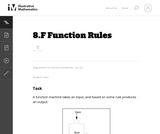Unrestricted Use
CC BY
Rating
0.0 stars

This task can be played as a game where students have to guess the rule and the instructor gives more and more input output pairs. Giving only three input output pairs might not be enough to clarify the rule.

Material Type:
Activity/Lab
Provider:
Illustrative Mathematics
Provider Set:
Illustrative Mathematics
Author:
Illustrative Mathematics
10/30/2013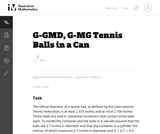Unrestricted Use
CC BY
Rating
0.0 stars

This task is inspired by the derivation of the volume formula for the sphere. If a sphere of radius 1 is enclosed in a cylinder of radius 1 and height 2, then the volume not occupied by the sphere is equal to the volume of a Ňdouble-naped coneÓ with vertex at the center of the sphere and bases equal to the bases of the cylinder.

Material Type:
Activity/Lab
Provider:
Illustrative Mathematics
Provider Set:
Illustrative Mathematics
Author:
Illustrative Mathematics